×#### Thank you for registering.

One of our academic counsellors will contact you within 1 working day.

Click to Chat

1800-1023-196

+91-120-4616500

CART 0

• 0

MY CART (5)

Use Coupon: CART20 and get 20% off on all online Study Material

ITEM
DETAILS
MRP
DISCOUNT
FINAL PRICE
Total Price: Rs.

There are no items in this cart.
Continue Shopping• Complete JEE Main/Advanced Course and Test Series
• OFFERED PRICE: Rs. 15,900
• View Details

```Congruence Exercise 16.5

Question: 1

In each of the following pairs of right triangles, the measures of some part are indicated alongside. State by the application of RHS congruence conditions which are congruent, and also state each result in symbolic form.

Solution:

i)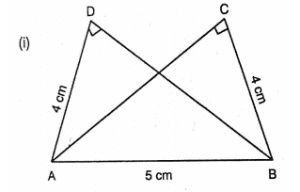AD = BC and hyp AB = hyp AB

Therefore, by RHS ΔADB ≅ ΔACB

ii)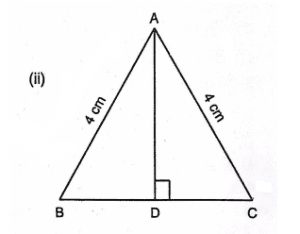hyp AC = hyp AB (Given)

∠ADB = 180° – 90° = 90°

iii)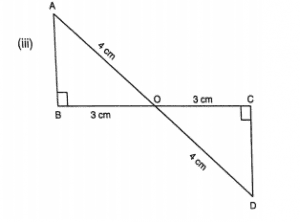hyp AO = hyp DOBO = CO ∠B = ∠C = 90°

Therefore, by RHS, ΔAOB≅ΔDOC

iv)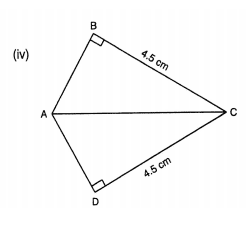Hyp A = Hyp CABC = DC ∠ABC = ∠ADC = 90°

Therefore, by RHS, ΔABC ≅ ΔADC

v)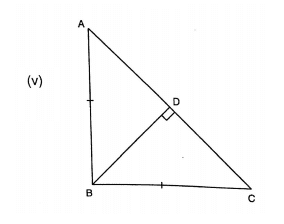BD = DB Hyp AB = Hyp BC, as per the given figure,

∠BDA + ∠BDC = 180°

∠BDA + 90° = 180°

∠BDA= 180°- 90° = 90°

∠BDA = ∠BDC = 90°

Therefore, by RHS, ΔABD ≅ ΔCBD

Question: 2

Δ ABC is isosceles with AB = AC. AD is the altitude from A on BC.

i) Is ΔABD ≅ ΔACD?

(ii) State the pairs of matching parts you have used to answer (i).

(iii) Is it true to say that BD = DC?

Solution:

(i) Yes, ΔABD ≅ ΔACD by RHS congruence condition.

(ii) We have used Hyp AB = Hyp AC

(iii)Yes, it is true to say that BD = DC (c.p.c.t) since we have already proved that the two triangles are congruent.

Question: 3

ΔABC is isosceles with AB = AC. Also. AD ⊥ BC meeting BC in D. Are the two triangles ABD and ACD congruent? State in symbolic form. Which congruence condition do you use? Which side of ADC equals BD? Which angle of Δ ADC equals ∠B?

Solution:

We have AB = AC            …… (i)

Therefore, from (i), (ii) and (iii), by RHS congruence condition, ΔABD ≅ ΔACD, the triangles are congruent.

Therefore, BD = CD.

And ∠ABD = ∠ACD (c.p.c.t)

Question: 4

Draw a right triangle ABC. Use RHS condition to construct another triangle congruent to it.

Solution:Consider

Δ ABC with ∠B as right angle.

We now construct another triangle on base BC, such that ∠C is a right angle and AB = DC

Also, BC = CB

Therefore, BC = CB

Therefore by RHS, ΔABC ≅ ΔDCB

Question: 5

In figure, BD and CE are altitudes of Δ ABC and BD = CE.

(i) Is ΔBCD ≅ ΔCBE?

(ii) State the three pairs or matching parts you have used to answer (i)Solution:

(i) Yes, ΔBCD ≅ ΔCBE by RHS congruence condition.

(ii) We have used hyp BC = hyp CB

BD = CE (Given in question)

And ∠BDC = ∠CBE = 90o
```### Course Features

• 728 Video Lectures
• Revision Notes
• Previous Year Papers
• Mind Map
• Study Planner
• NCERT Solutions
• Discussion Forum
• Test paper with Video Solution